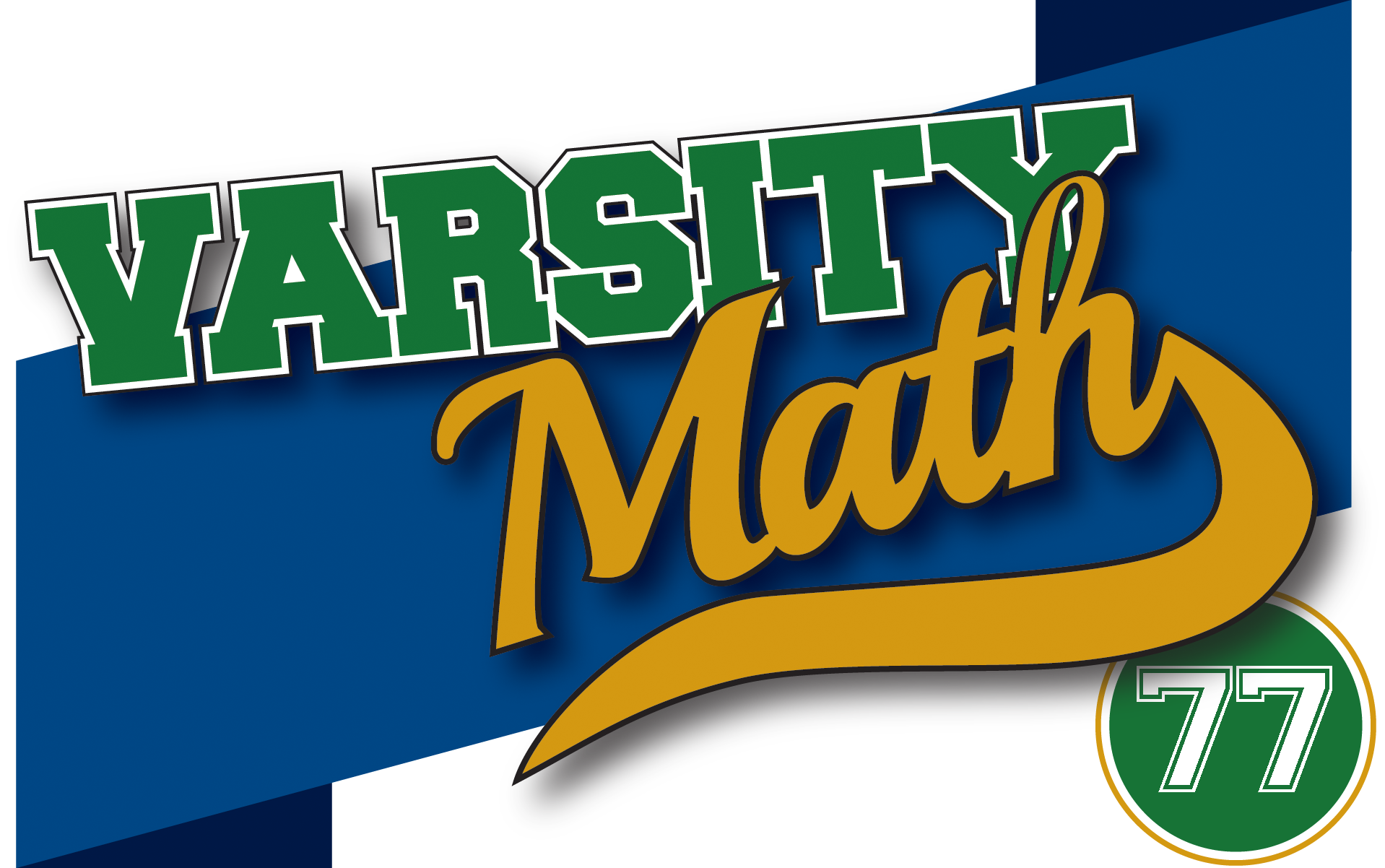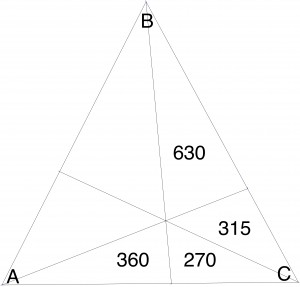## ________________

This past Tuesday, Coach Newton was honored as a special guest at the National Museum of Mathematics’ MoMath Masters tournament, held in Tribeca (the TRIangular area BElow CAnal street) in Manhattan. The students congratulate the coach by posing some problems about areas related to triangles.

## ________________### Trial Squares

Jess experiments with two different ways that a square can be inscribed into the same isosceles right triangle: In one way, two of the square’s sides lie exactly along the two legs of the triangle, and one of its vertices just touches the hypotenuse. The other way, one of its sides lies exactly along the hypotenuse, and two of its vertices just touch the legs of the triangle. For the triangle under consideration, Jess notices that the area of the larger of these two squares is 576.

What is the area of the smaller of the two squares?### Four of Six

Triangle ABC is divided into six smaller triangles by three line segments from the vertices, all through the same interior point, as shown. Some of the areas of the smaller triangles are given in the diagram.

What is the area of triangle ABC?

## Solutions to week 76

Unleash Your Inner Rectangle.The accompanying figure shows quadrilateral ABCD with midpoint rectangle EFGH, as well as point K, the intersection of the two diagonals AC and BD. Since AH is half of AD and AE is half of AB, triangle AHE is similar to triangle ADB. That means that angle AHE is equal to angle ADB, so lines HE and BD are parallel. Reasoning similarly about triangles BEF and BAC, we conclude that lines EF and AC are parallel. Since HE and EF are adjacent sides of a rectangle, they are perpendicular, so BD and AC are perpendicular as well. In particular, triangle ABK is a right triangle; since one acute angle CAB is given as 17 degrees, the other one ABD is 73 degrees.

Pent-up-gon.The diagram shows pentagon ABCDE with midpoint regular pentagon FGHJK, as well as auxiliary lines AC, CE, EB, BD, and DA. Reasoning about similar triangles just as in the previous problem, we see that these auxiliary lines are each parallel to, and twice as long as, FG, HJ, KF, GH, and JK, respectively. Thus all of the auxiliary lines are equal, and given they make the same angles to each other as the sides of the regular pentagon FGHJK do, the entire dashed figure is a regular pentagram. But the vertices of a regular pentagram form a regular pentagon, so BC = CD, and the ratio asked for is one.

## Recent Weeks

Links to all of the puzzles and solutions are on the Complete Varsity Math page.

Come back next week for answers and more puzzles.

[asciimathsf]